• 设计一个巴特沃斯模拟低通滤波器，要求通带截止频率 ，通带最大衰减 ，阻带截止频率 ，阻带最小衰减 。要求绘出滤波器的幅频特性曲线。
• 给定的技术指标是：已知通带截止频率fp=5kHz，通带最大衰减Wp=2dB，阻带截止频率fs=12kHz，阻带最小衰减Ws=30dB，按照以上技术指标设计巴特沃斯低通滤波器。程序如下：>> Wp=2*pi*5000; %通带截止角频率>> Ws=2*pi*...
给定的技术指标是：已知通带截止频率fp=5kHz，通带最大衰减Wp=2dB，阻带截止频率fs=12kHz，阻带最小衰减Ws=30dB，按照以上技术指标设计巴特沃斯低通滤波器。
程序如下：
>> Wp=2*pi*5000;                          %通带截止角频率>> Ws=2*pi*12000;                         %阻带截止角频率>> Rp=2;                                  %通带最大衰减>> Rs=30;                                 %阻带最小衰减>> [n,Wn] = buttord(Wp,Ws,Rp,Rs,'s');     %求巴特沃斯阶数和3db截止角频率>> [b,a] = butter(n,Wn,'s');              %求传递函数>> [z,p,k] = butter(n,Wn,'s');            %求零极点及增益>> w=linspace(1,15000)*2*pi;             >> H =freqs(b,a,w);                       %频率响应>> magH=abs(H);                           %频率响应的幅度>> phaH=unwrap(angle(H));                 %频率响应的相位(平滑处理)    >> plot(w/(2*pi),20*log10(magH));         %频率响应的幅度的曲线图                >> title('巴特沃斯低通滤波器的幅频特性');  >> xlabel('频率/Hz');                       >> ylabel('幅度/db')       
展开全文plot fp
• 1.低通滤波器 低通滤波是将频域图像中的高频部分滤除而通过低频部分。图像的边缘和噪声对应于频域图像中的高频部分，而低通滤波的作用即是减弱这部分的能量，从而达到图像平滑去噪的目的。 2.理想低通滤波器 最简单...
1.低通滤波器 低通滤波是将频域图像中的高频部分滤除而通过低频部分。图像的边缘和噪声对应于频域图像中的高频部分，而低通滤波的作用即是减弱这部分的能量，从而达到图像平滑去噪的目的。
2.理想低通滤波器 最简单的低通滤波器是理想低通滤波器，基本思想是给定一个频率阈值，将高于该阈值的所有部分设置为0，而低于该频率的部分保持不变。
理想是指该滤波器不能用电子元器件来实现，但是可以通过计算机来模拟。
在VTK中定义了理想低通滤波器，下面我们来看下怎么使用该滤波器来对图像进行低通滤波:
//理想低通滤波器
#include <vtkSmartPointer.h>
#include <vtkImageFFT.h>
#include <vtkImageIdealLowPass.h>
#include <vtkImageData.h>
#include <vtkImageRFFT.h>
#include <vtkImageCast.h>
#include <vtkImageExtractComponents.h>
#include <vtkImageActor.h>
#include <vtkRenderer.h>
#include <vtkRenderWindow.h>
#include <vtkRenderWindowInteractor.h>

int main()
{

vtkSmartPointer<vtkImageFFT> fftFilter = vtkSmartPointer<vtkImageFFT>::New();
fftFilter->Update();

vtkSmartPointer<vtkImageIdealLowPass> lowPassFilter = vtkSmartPointer<vtkImageIdealLowPass>::New();
lowPassFilter->SetInputConnection(fftFilter->GetOutputPort());
lowPassFilter->SetXCutOff(0.05); //设置x\y方向上的截断频率
lowPassFilter->SetYCutOff(0.05);
lowPassFilter->Update();

vtkSmartPointer<vtkImageRFFT> rfftFilter = vtkSmartPointer<vtkImageRFFT>::New();//将处理后的频域图像转换至空域图像，
rfftFilter->SetInputConnection(lowPassFilter->GetOutputPort());              //注意：转换后的图像每个像素值都是一个复数；
rfftFilter->Update();

vtkSmartPointer<vtkImageExtractComponents> ifftExtractReal = vtkSmartPointer<vtkImageExtractComponents>::New();
ifftExtractReal->SetInputConnection(rfftFilter->GetOutputPort());
ifftExtractReal->SetComponents(0);//提取实部分量

vtkSmartPointer<vtkImageCast> castFilter = vtkSmartPointer<vtkImageCast>::New();//数据类型转换为unsigned char类型
castFilter->SetInputConnection(ifftExtractReal->GetOutputPort());
castFilter->SetOutputScalarTypeToUnsignedChar();
castFilter->Update();

vtkSmartPointer<vtkImageActor> originalActor = vtkSmartPointer<vtkImageActor>::New();

vtkSmartPointer<vtkImageActor> erodedActor = vtkSmartPointer<vtkImageActor>::New();
erodedActor->SetInputData(castFilter->GetOutput());

double leftViewport = { 0.0, 0.0, 0.5, 1.0 };
double rightViewport = { 0.5, 0.0, 1.0, 1.0 };
vtkSmartPointer<vtkRenderer> leftRenderer = vtkSmartPointer<vtkRenderer>::New();
leftRenderer->ResetCamera();
leftRenderer->SetViewport(leftViewport);
leftRenderer->SetBackground(1.0, 0, 0);

vtkSmartPointer<vtkRenderer> rightRenderer = vtkSmartPointer<vtkRenderer>::New();
rightRenderer->SetViewport(rightViewport);
rightRenderer->ResetCamera();
rightRenderer->SetBackground(1.0, 1.0, 1.0);

vtkSmartPointer<vtkRenderWindow> renderWindow = vtkSmartPointer<vtkRenderWindow>::New();
renderWindow->SetSize(540, 320);
renderWindow->SetWindowName("Frequency_IdealLowPassFilter");

vtkSmartPointer<vtkRenderWindowInteractor> interactor = vtkSmartPointer<vtkRenderWindowInteractor>::New();
interactor->SetRenderWindow(renderWindow);
renderWindow->Render();
interactor->Start();

return 0;
}
运行结果如下：从结果看，在过滤掉图像的高频部分后，图像变得模糊，丢失了许多细节，另外还可以看到图像会存在一定的振铃效应，这也是理想低通滤波的特点。
首先读入一副图像，通过vtkImageFFT将图像转换到频域空间。vtkImageIdealLowPass对频域图像做理想低通滤波，需要用户设置每个方向的截断频率，相应的设置函数SetXCutOff()和SetYCutOff()。执行完毕后，需要通过vtkImageRFFT将处理后的频域图像转换至空域图像。需要注意的是，转换后的图像每个像素都是一个复数，需要vtkImageExtractComponents将该图像的第一个分量提出出来显示，否则图像不能正确显示。由于傅里叶变换输入输出的数据类型都是double，为了方便显示，还需要将其转换为Unsigned char类型，这里vtkImageCast负责类型转换。3.巴特沃兹低通滤波器
在实际中经常使用的是巴特沃斯滤波器。巴特沃斯滤波器对应的转移函数（可以看做是一个系数矩阵）是：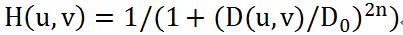其中D(u,v)表示频域点(u,v)到频域图像原点的距离，称为截止频率，当D(u,v) = 时，H(u,v)=0.5，即对应的频域能量将为原来的一半。因为巴特沃斯低通滤波器在高低频间的过渡平滑，因此不会出现明显的振铃效应。VTK中实现巴特沃斯低通滤波器的类是vtkImageButterworthLowPass.
/***********************巴特沃兹低通滤波器*****************************************/
#include <vtkSmartPointer.h>
#include <vtkImageFFT.h>
#include <vtkImageButterworthLowPass.h>
#include <vtkImageRFFT.h>
#include <vtkImageExtractComponents.h>
#include <vtkImageCast.h>
#include <vtkImageActor.h>
#include <vtkRenderer.h>
#include <vtkRenderWindow.h>
#include <vtkRenderWindowInteractor.h>
#include <vtkInteractorStyleImage.h>

int main()
{

vtkSmartPointer<vtkImageFFT> fftFilter = vtkSmartPointer<vtkImageFFT>::New();
fftFilter->Update();

vtkSmartPointer<vtkImageButterworthLowPass> lowPassFilter = vtkSmartPointer<vtkImageButterworthLowPass>::New();
lowPassFilter->SetInputConnection(fftFilter->GetOutputPort());
lowPassFilter->SetXCutOff(0.05);
lowPassFilter->SetYCutOff(0.05);
lowPassFilter->Update();

vtkSmartPointer<vtkImageRFFT> rfftFilter = vtkSmartPointer<vtkImageRFFT>::New();
rfftFilter->SetInputConnection(lowPassFilter->GetOutputPort());
rfftFilter->Update();

vtkSmartPointer<vtkImageExtractComponents> ifftExtractReal = vtkSmartPointer<vtkImageExtractComponents>::New();
ifftExtractReal->SetInputConnection(rfftFilter->GetOutputPort());
ifftExtractReal->SetComponents(0);

vtkSmartPointer<vtkImageCast> castFilter = vtkSmartPointer<vtkImageCast>::New();
castFilter->SetInputConnection(ifftExtractReal->GetOutputPort());
castFilter->SetOutputScalarTypeToUnsignedChar();
castFilter->Update();

vtkSmartPointer<vtkImageActor> originalActor = vtkSmartPointer<vtkImageActor>::New();

vtkSmartPointer<vtkImageActor> erodedActor = vtkSmartPointer<vtkImageActor>::New();
erodedActor->SetInputData(castFilter->GetOutput());
///
double leftViewport = { 0.0, 0.0, 0.5, 1.0 };
double rightViewport = { 0.5, 0.0, 1.0, 1.0 };
vtkSmartPointer<vtkRenderer> leftRenderer =	vtkSmartPointer<vtkRenderer>::New();
leftRenderer->SetViewport(leftViewport);
leftRenderer->SetBackground(1.0, 1.0, 1.0);
leftRenderer->ResetCamera();

vtkSmartPointer<vtkRenderer> rightRenderer = vtkSmartPointer<vtkRenderer>::New();
rightRenderer->SetViewport(rightViewport);
rightRenderer->SetBackground(1.0, 1.0, 1.0);
rightRenderer->ResetCamera();

vtkSmartPointer<vtkRenderWindow> rw = vtkSmartPointer<vtkRenderWindow>::New();
rw->SetSize(640, 320);
rw->SetWindowName("ButterworthLowPassExample");

vtkSmartPointer<vtkRenderWindowInteractor> rwi = vtkSmartPointer<vtkRenderWindowInteractor>::New();
vtkSmartPointer<vtkInteractorStyleImage> style = vtkSmartPointer<vtkInteractorStyleImage>::New();
rwi->SetInteractorStyle(style);
rwi->SetRenderWindow(rw);
rwi->Start();

return 0;
}
运行结果如下：vtkImageButterworthLowPass与理想低通滤波器的使用一样。为了便于比较，这里设置X和Y方向的截止频率时，与理想低通滤波器设置一致，从结果来看，巴特沃斯低通滤波器产生的图像更为平滑，不会出现振铃现象。

参考资料：
1.《The Visualization Toolkit – AnObject-Oriented Approach To 3D Graphics (4th Edition)》 2. 张晓东, 罗火灵. VTK图形图像开发进阶[M]. 机械工业出版社, 2015.
所用软件：vtk7.0+visual studio 2013
注：此文知识学习笔记，仅记录完整程序和实现结果，具体原理参见：
https://blog.csdn.net/www_doling_net/article/details/8541534
https://blog.csdn.net/shenziheng1/article/category/6114053/4
展开全文• 1 低通滤波器性能指标 低通滤波器的主要性能指标有四个： 通带截止频率：通带内幅值下降至一定程度时对应的频率，单位为：Hz或角频率 阻带截止频率：阻带内幅值高于一定程度时对应的频率，单位为：Hz或角频率 通带...

Hello，欢迎来到我的博客~~~

1 低通滤波器性能指标
低通滤波器的主要性能指标有四个：
通带截止频率：通带内幅值下降至一定程度时对应的频率，单位为：Hz或角频率阻带截止频率：阻带内幅值高于一定程度时对应的频率，单位为：Hz或角频率通带纹波（衰减）：通带中最大幅值和最小幅值之间的差值，单位为：dB阻带衰减：阻带中最大幅值和通带最大幅值的差值，单位为：dB

幅值单位：dB，计算方式：

20

log

⁡

10

A

20\log_{10}A

示例：
假如幅值响应是100，那么相当于

20

log

⁡

10

100

=

40

20\log_{10}100=40

dB假如通带最大幅值是100，阻带最大幅值是10，那么阻带衰减就是

20

log

⁡

10

10

−

20

log

⁡

10

100

=

−

20

20\log_{10}10 - 20\log_{10}100=-20

dB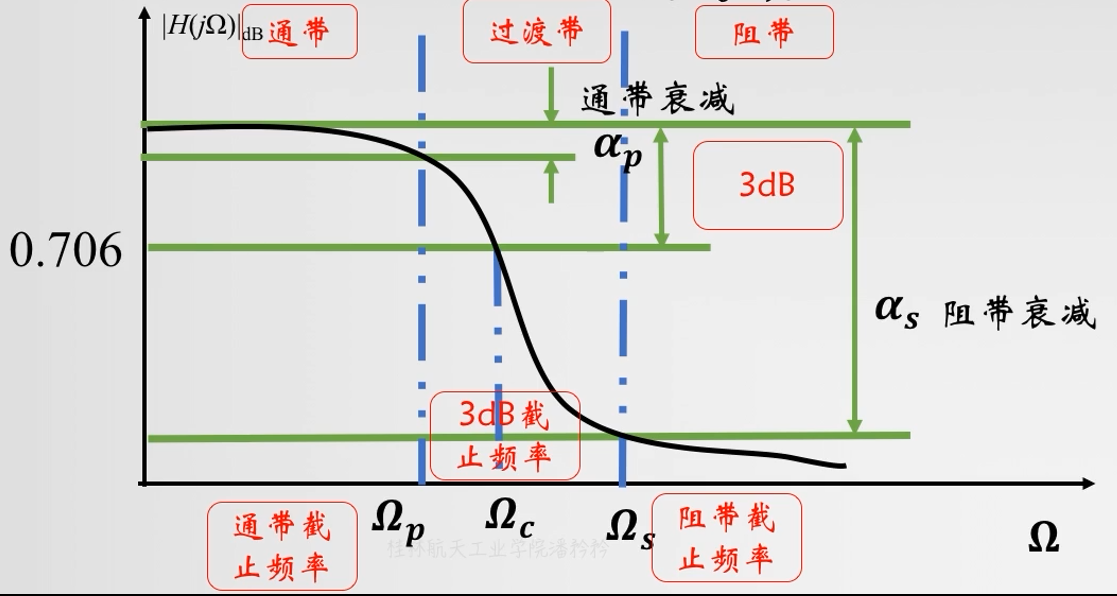2 巴特沃斯模拟低通滤波器设计步骤
2.1 巴特沃斯低通滤波器的传递函数
极点形式：

H

(

s

)

=

G

0

∏

k

=

1

n

1

s

−

ω

c

s

k

,

s

k

=

exp

⁡

j

(

2

k

+

n

−

1

)

2

n

,

k

=

1

,

2

,

3

,

.

.

.

,

n

H(s)=G_0\prod_{k=1}^n \frac{1}{s-\omega_cs_k},\ s_k=\exp\frac{j(2k+n-1)}{2n}, \ k=1,2,3,...,n

其中，

ω

c

\omega_c

就是-3dB截止频率，

n

n

是滤波器阶数，

G

0

G_0

是直流增益，一般取1。 多项式形式：

H

(

s

)

=

G

0

∑

k

=

0

n

a

k

(

s

/

ω

c

)

k

,

a

k

=

∏

μ

=

1

k

cos

⁡

(

(

μ

−

1

)

γ

)

sin

⁡

(

μ

γ

)

,

a

0

=

1

,

γ

=

π

2

n

,

k

=

1

,

2

,

3

,

.

.

.

,

n

H(s)=\frac{G_0}{\sum_{k=0}^n a_k({s}/{\omega_c})^k} ,\ a_k=\prod_{\mu=1}^{k}\frac{\cos((\mu-1)\gamma)}{\sin(\mu\gamma)},\ a_0=1, \ \gamma=\frac{\pi}{2n}, \ k=1,2,3,...,n

对应于不同阶数的滤波器，

s

k

s_k

和

a

k

a_k

的值都是可以事先计算好，然后查表得到的，如下示例：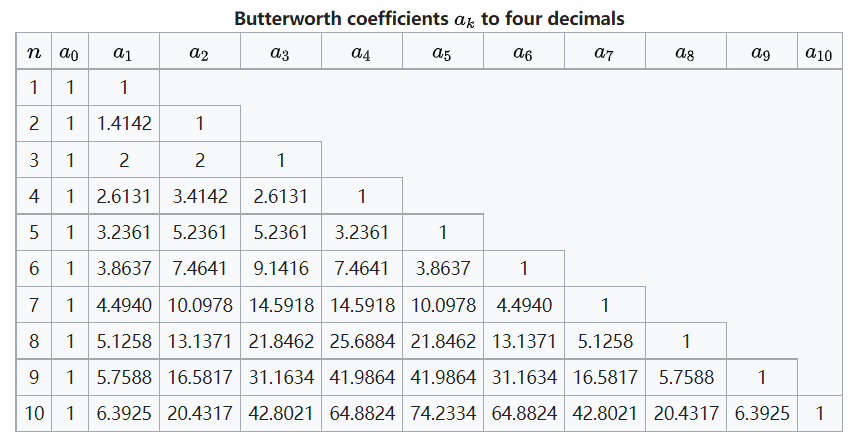2.2 频率响应
根据巴特沃斯滤波器的传递函数，可以推得其频率响应为：

∣

H

(

j

ω

)

∣

2

=

G

0

2

1

+

(

ω

ω

c

)

2

n

|H(j\omega)|^2=\frac{G_0^2}{1+(\frac{\omega}{\omega_c})^{2n}}

根据上述的频率响应，很容易分析得到当

ω

\omega

趋于0时，频率响应趋于

G

0

2

G_0^2

，当

ω

\omega

趋于无穷时，频率响应趋于0。

2.3 设计步骤
1. 给定通带截止频率

ω

p

\omega_p

，阻带截止频率

ω

s

\omega_s

，通带纹波

α

s

\alpha_s

和阻带衰减

α

p

\alpha_p

，计算滤波器阶数N

计算两个辅助变量：

k

s

p

=

1

0

0.1

α

s

−

1

1

0

0.1

α

p

−

1

k_{sp}=\sqrt{\frac{10^{0.1\alpha_s}-1}{10^{0.1\alpha_p}-1}}

,

λ

s

p

=

ω

s

ω

p

\lambda_{sp}=\frac{\omega_s}{\omega_p}

计算阶数（向上取整）：

N

=

lg

⁡

k

s

p

lg

⁡

λ

s

p

N=\frac{\lg{k_sp}}{\lg{\lambda_{sp}}}

2. 根据滤波器阶数，通过查表得到滤波器传递函数的系数

如上文所示，从表格中找出对应阶数滤波器的系数值：

a

k

a_k

3. 计算3 dB截止频率

ω

c

\omega_c

ω

c

=

ω

p

(

1

0

0.1

a

p

−

1

)

−

1

2

N

\omega_c=\omega_p(10^{0.1a_p}-1)^{-\frac{1}{2N}}

4. 代入系数和

ω

c

\omega_c

，得到最终的滤波器传递函数

H

(

s

)

=

G

0

∑

k

=

0

n

a

k

(

s

/

ω

c

)

k

H(s)=\frac{G_0}{\sum_{k=0}^n a_k({s}/{\omega_c})^k}

3 巴特沃斯数字低通滤波器设计步骤（IIR实现）
选择一个归一化的模拟滤波器（确定巴特沃斯低通滤波器的阶数）确定数字滤波器的3 dB截止频率利用公式计算模拟滤波器的3 dB截止频率

f

a

=

f

s

π

tan

⁡

π

f

d

f

s

f_a=\frac{f_s}{\pi}\tan{\frac{\pi f_d}{f_s}}

f

s

f_s

是采样频率，

f

d

f_d

是数字滤波器截止频率

将模拟截止频率

ω

c

=

2

π

f

a

\omega_c=2\pi f_a

带入模拟滤波器传递函数

H

(

s

)

H(s)

用双线性变换，把模拟滤波器传递函数中的

s

s

替换为

z

z

，得到

H

(

z

)

H(z)

双线性变换：

s

=

2

f

s

(

z

−

1

z

+

1

)

s=2f_s(\frac{z-1}{z+1})

4 巴特沃斯高通、带通、带阻数字滤波器的设计
要设计高通、带通、带阻等数字滤波器，有两种思路。
低通模拟滤波器 =》=》高通、带通、带阻模拟滤波器 =》=》高通、带通、带阻数字滤波器低通模拟滤波器 =》=》高通、带通、带阻数字滤波器
这里主要介绍的是第二种思想，方法如下图所示：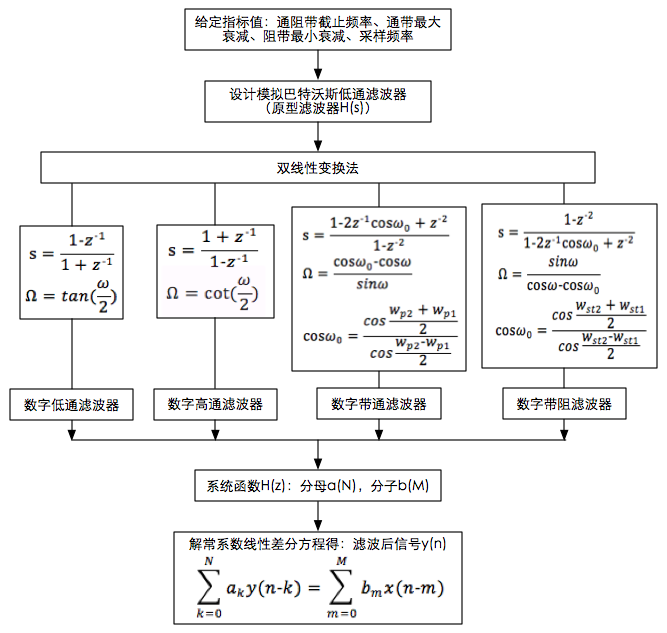4.1 变量说明

ω

=

2

π

f

p

f

s

\omega=\frac{2\pi f_p}{f_s}

，其中，

f

p

f_p

是数字通带截止频率

Ω

=

2

π

F

p

f

s

\Omega=\frac{2\pi F_p}{f_s}

，其中，

F

p

F_p

是模拟通带截止频率（注意这里的符号和上文有差异，不要混淆）

ω

p

1

=

2

π

f

p

1

f

s

\omega_{p1}=\frac{2\pi f_{p1}}{f_s}

，其中，

f

p

1

f_{p1}

是数字下通带截止频率（带通滤波器）

ω

p

2

=

2

π

f

p

2

f

s

\omega_{p2}=\frac{2\pi f_{p2}}{f_s}

，其中，

f

p

2

f_{p2}

是数字上通带截止频率（带通滤波器）

ω

s

t

1

=

2

π

f

s

t

1

f

s

\omega_{st1}=\frac{2\pi f_{st1}}{f_s}

，其中，

f

s

t

1

f_{st1}

是数字下阻带截止频率（带阻滤波器）

ω

s

t

2

=

2

π

f

s

t

2

f

s

\omega_{st2}=\frac{2\pi f_{st2}}{f_s}

，其中，

f

s

t

2

f_{st2}

是数字上阻带截止频率（带阻滤波器）
4.2 设计步骤
根据上图，设计步骤可以描述如下：
选定巴特沃斯滤波器的阶数，可以得到一个归一化的巴特沃斯低通滤波器，形式如下：

H

(

p

)

=

1

∑

k

=

0

N

a

k

p

k

H(p)=\frac{1}{\sum_{k=0}^N a_kp^k}

针对不同的滤波器形式，利用图中公式计算出

Ω

\Omega

，并在

H

(

p

)

H(p)

中带入

p

=

s

Ω

p=\frac{s}{\Omega}

，得到

H

(

s

)

H(s)

针对不同的滤波器形式，利用图中公式，将

H

(

s

)

H(s)

公式中的

s

s

用

z

z

变量替换，得到

H

(

z

)

H(z)

注意事项： 上图中对应低通、高通、带通、带阻都有

s

s

和

Ω

\Omega

的计算方法 需要注意的是， 对于低通和高通而言，

ω

\omega

一般指的都是通带

3

3

dB截止频率 对于带通和带阻而言，

ω

\omega

一般指的都是上通带或上阻带

3

3

dB截止频率

4.3 设计示例
4.3.1 巴特沃斯数字高通滤波器
给定条件：滤波器阶数为1，数字滤波器通带截止频率为30 Hz，采样频率为100 Hz 第一步：查表，得到归一化巴特沃斯低通滤波器形式：

H

(

p

)

=

1

p

+

1

H(p)=\frac{1}{p+1}

第二步：计算

ω

\omega

和

Ω

\Omega

，在

H

(

p

)

H(p)

中带入

p

=

s

Ω

p=\frac{s}{\Omega}

ω

=

2

π

∗

30

100

=

0.6

π

,

Ω

=

cot

⁡

ω

2

=

0.7265

\omega=\frac{2\pi*30}{100}=0.6\pi,\ \Omega=\cot{\frac{\omega}{2}}=0.7265

H

(

s

)

=

0.7265

s

+

0.7265

H(s)=\frac{0.7265}{s+0.7265}

第三步：将

H

(

s

)

H(s)

公式中的

s

s

用

z

z

变量替换

H

(

z

)

=

0.7265

z

−

1

z

+

1

+

0.7265

=

0.7265

z

−

0.7265

1.7265

z

+

0.2735

=

0.4208

z

−

0.4208

z

+

0.1584

H(z)=\frac{0.7265}{\frac{z-1}{z+1}+0.7265}=\frac{0.7265z-0.7265}{1.7265z+0.2735}=\frac{0.4208z-0.4208}{z+0.1584}

以上是设计得到的巴特沃斯数字高通滤波器，利用Matlab可以验算结果，和公式计算的完全一致。

Matlab命令： fc = 30; fs = 100; [a,b]=butter(1, fc/(fs/2), ‘high’) 结果： a = [0.4208, -0.4208] % 分子多项式系数 b = [1.0000, 0.1584] % 分母多项式系数

4.3.2 巴特沃斯数字带阻滤波器
给定条件：滤波器阶数为2，数字滤波器上阻带截止频率为15 Hz，下阻带截止频率为10 Hz，采样频率为100 Hz 第一步：查表，得到归一化巴特沃斯低通滤波器形式：

H

(

p

)

=

1

p

2

+

1.4142

p

+

1

H(p)=\frac{1}{p^2+1.4142p+1}

第二步：计算

ω

\omega

，

ω

s

t

1

\omega_{st1}

，

ω

s

t

2

\omega_{st2}

和

Ω

\Omega

，在

H

(

p

)

H(p)

中带入

p

=

s

Ω

p=\frac{s}{\Omega}

ω

s

t

1

=

2

π

∗

10

100

=

0.2

π

,

ω

s

t

2

=

2

π

∗

15

100

=

0.3

π

,

cos

⁡

ω

0

=

cos

⁡

ω

s

t

2

+

ω

s

t

1

2

cos

⁡

ω

s

t

2

−

ω

s

t

1

2

=

cos

⁡

0.3

π

+

0.2

π

2

cos

⁡

0.3

π

−

0.2

π

2

=

0.7159

,

Ω

s

t

1

=

sin

⁡

ω

s

t

1

cos

⁡

ω

s

t

1

−

cos

⁡

ω

0

=

sin

⁡

0.2

π

cos

⁡

0.2

π

−

0.7159

=

6.3123

,

Ω

s

t

2

=

sin

⁡

ω

s

t

2

cos

⁡

ω

s

t

2

−

cos

⁡

ω

0

=

sin

⁡

0.3

π

cos

⁡

0.3

π

−

0.7159

=

−

6.3148

,

Ω

=

Ω

s

t

1

\begin{aligned} &\omega_{st1}=\frac{2\pi*10}{100}=0.2\pi, \ \omega_{st2}=\frac{2\pi*15}{100}=0.3\pi,\\ &\cos\omega_0=\frac{\cos\frac{\omega_{st2}+\omega_{st1}}{2}}{\cos\frac{\omega_{st2}-\omega_{st1}}{2}}=\frac{\cos\frac{0.3\pi+0.2\pi}{2}}{\cos\frac{0.3\pi-0.2\pi}{2}}=0.7159,\\ &\Omega_{st1}=\frac{\sin\omega_{st1}}{\cos\omega_{st1}-\cos\omega_0}=\frac{\sin0.2\pi}{\cos0.2\pi-0.7159}=6.3123,\\ &\Omega_{st2}=\frac{\sin\omega_{st2}}{\cos\omega_{st2}-\cos\omega_0}=\frac{\sin0.3\pi}{\cos0.3\pi-0.7159}=-6.3148,\\ &\Omega = \Omega_{st1} \end{aligned}

H

(

s

)

=

6.312

3

2

s

2

+

6.3123

s

+

6.312

3

2

=

39.8451

s

2

+

6.3123

s

+

39.8451

H(s)=\frac{6.3123^2}{s^2+6.3123s+6.3123^2}=\frac{39.8451}{s^2+6.3123s+39.8451}

第三步：将

H

(

s

)

H(s)

公式中的

s

s

用

z

z

变量替换

H

(

z

)

=

39.8451

(

z

2

−

1

z

2

−

2

cos

⁡

ω

0

z

+

1

)

2

+

6.3123

z

2

−

1

z

2

−

2

cos

⁡

ω

0

z

+

1

+

39.8451

=

39.8451

(

z

2

−

1.4318

z

+

1

)

2

(

z

2

−

1

)

2

+

6.3123

(

z

2

−

1

)

(

z

2

−

1.4318

z

+

1

)

+

39.8451

(

z

2

−

1.4318

z

+

1

)

2

=

39.8451

(

z

4

−

2.8636

z

3

+

4.0501

z

2

−

2.8636

z

+

1

)

47.1574

z

4

−

123.1384

z

3

+

159.3747

z

2

−

105.0625

z

+

34.5328

=

0.8449

z

4

−

2.4196

z

3

+

3.4221

z

2

−

2.4196

z

+

0.8449

z

4

−

2.6112

z

3

+

3.3796

z

2

−

2.2279

z

+

0.7323

\begin{aligned} H(z)&=\frac{39.8451}{(\frac{z^2-1}{z^2-2\cos\omega_0z+1})^2+6.3123\frac{z^2-1}{z^2-2\cos\omega_0z+1}+39.8451}\\ &=\frac{39.8451(z^2-1.4318z+1)^2}{(z^2-1)^2+6.3123(z^2-1)(z^2-1.4318z+1)+39.8451(z^2-1.4318z+1)^2}\\ &=\frac{39.8451(z^4 - 2.8636z^3 + 4.0501z^2 - 2.8636z + 1)}{47.1574z^4 - 123.1384z^3 + 159.3747z^2 - 105.0625z+ 34.5328}\\ &=\frac{0.8449z^4 - 2.4196z^3 + 3.4221z^2 - 2.4196z + 0.8449}{z^4 - 2.6112z^3 + 3.3796z^2 - 2.2279z+ 0.7323}\\ \end{aligned}

以上是设计得到的巴特沃斯数字高通滤波器，利用Matlab可以验算结果，和公式计算的基本一致。

Matlab命令： fst1 = 10; fst2 = 15; fs = 100; [a,b]=butter(2,[fst1/(fs/2),fst2/(fs/2)],‘stop’) 结果： a = [0.8006, -2.2926, 3.2425, -2.2926, 0.8006] % 分子多项式系数 b = [1.0000, -2.5494, 3.2024, -2.0359, 0.6414] % 分母多项式系数

5 参考资料
参考链接：从模拟滤波器到数字滤波器 数字信号处理公式变程序（四）——巴特沃斯滤波器（上） 数字信号处理教程（超浓缩版）
展开全文• 基于Matlab巴特沃斯低通滤波器的设计谢继杨(成都理工大学工程技术学院，四川乐山，614000)摘要：现如今已经有相当成熟的技术去模拟滤波器，人们为了更加深入的理解巴特沃斯滤波器，于是巴特沃斯模拟滤波器便基于...

基于Matlab巴特沃斯低通滤波器的设计
谢继杨
(成都理工大学工程技术学院，四川乐山，614000)
摘要：现如今已经有相当成熟的技术去模拟滤波器，人们为了更加深入的理解巴特沃斯滤波器，于是巴特沃斯模拟滤波器便基于matlab来编程。同时几种模拟滤波器将被我们用于比较。
关键词：MATLAB；巴特沃斯低通滤波器；相关滤波器的比较
Design of Butterworth low pass filter based onMatlab
ZuozheXiejiyang
(TheEngineering&TechnicalCollege of Chengdu University of technology,Leshan,Sichuan614000)
Abstract:Nowadays, there are quite mature technologies to simulate filters. In order to have a deeper understanding ofbutterworthfilters,butterworthanalog filters are programmed based on MATLAB. At the same time several analog filters will be used for comparison.
Keywords:MATLAB；Butterworth low pass filter；Comparison of correlation filters
1.引言：
MATLAB属于科学计算软件的一种，在工程应用领域用于分析设计和复杂计算，方便的使用，简洁的输入，高效的运算，丰富的内容是它的代名词，并且编写后可作函数文件的方式储存，调用相当简单。滤波器这种电子装置可以在有用频率信号通过的同时衰减无用频率。在频率高于一个确定数值的电路中，滤波器便起到了抑制作用。所以，巴特沃斯滤波器能使用matlab来高速便捷的编程模拟。
2正文：
2.1巴特沃斯滤波器的原理
在现代各式各样的滤波器设计方法中，巴特沃斯型滤波器是最为为人知晓的，之所以被推广应用的原因，是因为它很容易就被设计，性能优点比起缺点更为明显，元件Q值在滤波器中属于低水平，因此很好制造还能达到设计标准。其最为明显的地方当属于在通频带那最为平滑的频率响应曲线。
要想实现改变截止频率，就必须先将还没设计的滤波器截止频率比上基准滤波器的截止频率，使用计算出的比值M去比上这当中所有的元件值。其中M的计算公式为；M=还未设计滤波器的截止频率/基准滤波器的截止频率。
关于变换特征阻抗，要将待设计的滤波器的特征阻抗去除以基准滤波器的特征阻抗，得到比值K，接下来用K去与该滤波器中的所有的电容元件值相比以及与基准滤波器中所有的电感元件值相乘，便可实现。
2.2巴特沃斯滤波器的优点
在通频带内最大程度的平坦，不起伏，在阻频带则慢慢下降至零是频率响应的特点。从一个边界角频率开始角频率逐渐增加振幅随之减少并趋向负无穷则是在振幅的对数对角频率的波特图特点。
每种滤波器在高阶与低阶呈现的振幅对角频率不同，巴特沃斯滤波器不一样的地方便是阶数、振幅对角频率保持了同样形状，只是在阻频带振幅衰减速度与阶数成正比。最为直观的表现为一阶巴特沃斯滤波器的衰减率六分贝每倍频，二阶巴特沃斯滤波器衰减率十二分贝每倍频，三阶巴特沃斯的衰减率十八分贝每倍频，特别指出在一阶时每十倍频是二十分贝。
类比于切比雪夫滤波器，巴特沃斯滤波器有高通，低通，带通，带阻等多种滤波器分类设计。平稳的幅频特点，较为漫长的过渡带，让它在通频带中容易在过渡带失真，在仿真时第一周期往往会出现失真，随之往后的幅频特性越来越好。
2.3多种滤波器的比较
Bessel滤波器在线性过滤器中具有最为平坦的群延迟以及最为平坦的幅度和相位响应。因此在音频天桥系统中使用最为常用。每到模拟Bessel滤波器时恒定的群延迟便出现在通频带内并几乎将其横跨，所以过滤的信号波形在通频带上被保留。几乎呈线性的相位响应则属于用户常常关注的区域——带通。所以Bessel滤波器用于减少非线性相位失真在所有IIR滤波器中这一固有特点。
切比雪夫这一滤波器的特点是频率响应幅度等波纹波动，发生在通带或阻带上，等波纹这一振幅特点在通带上。被称为切比雪夫Ⅰ型的滤波器则是在阻带内单调的；被称为切比雪夫Ⅱ型的滤波器则在通带才是单调的，在阻带内却是等波纹的。所以如何选用切比雪夫滤波器得看实际情况采取合适的型号针对性选用。
椭圆滤波器滤波器也就是常说的Cauer滤波器，在阶数相同的情况下通带和阻带波动最小，而且在通带和阻带波动相同，这也正是它被区别于巴特沃斯滤波器和切比雪夫滤波器的特点。如果从传递函数的角度出发，常数除以多项式是巴特沃斯和切比雪夫滤波器的特点，表现为衰减为无限大当处于无限大阻带时。而Cauer滤波器既有零点也有极点，产生等波纹时极点与零点在通带内，有限的传输零点在阻带内时产生了让过渡区减少的情况，所以方便获得的衰减曲线非常陡峭，这便形成了Cauer最大优势，不过有利还是会存在弊端，如此陡峭的衰减曲线正是通过通带和阻带的不稳定来换取的。而且由于传输函数较之巴特沃斯和切比雪夫滤波器更为繁琐，如果使用传统设计思路，设计程序，过程计算极为复杂。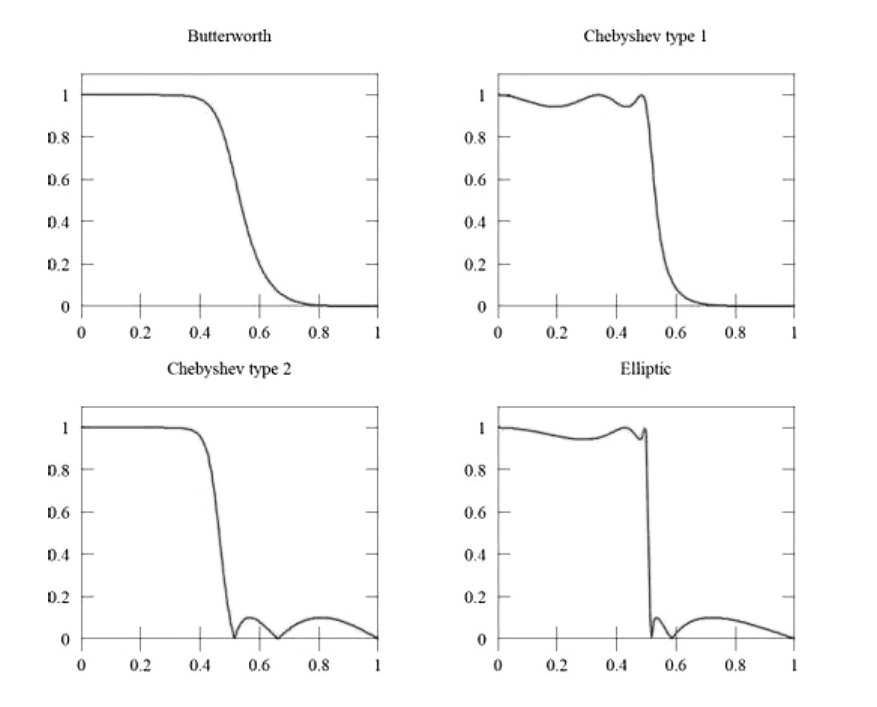巴特沃斯、切比雪夫、贝塞尔和椭圆滤波器比较
3实例
首先将巴特沃斯滤波器在matlab上模拟如下：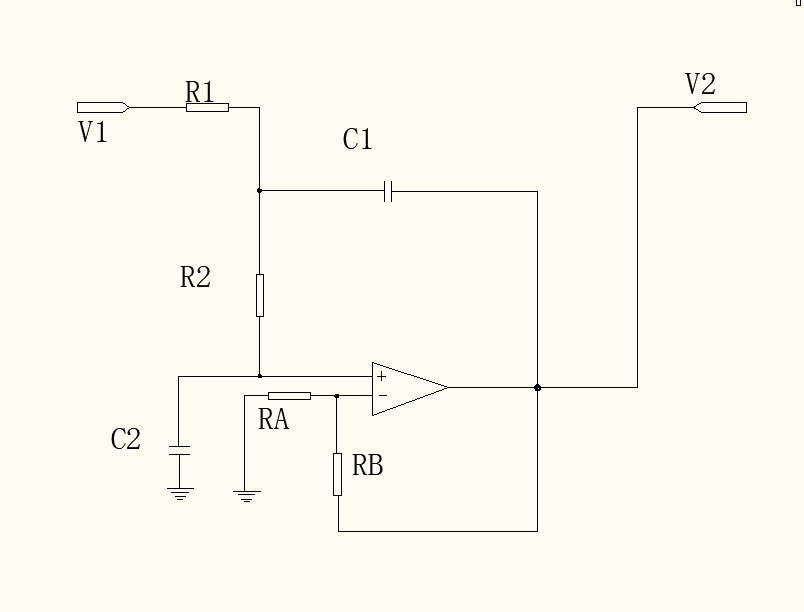图3.1.1二阶有源电路图
一、巴特沃斯低通滤波器可用公式表示为：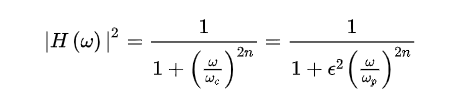图3.1.2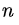=滤波器的阶数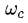=截止频率=振幅下降为-3分贝时的频率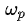=通频带边缘频率
二、为一个巴特沃斯低通滤波器设计技术标准：
通带截至频率fp=2.6kHz,
阻带截至频率fs=3.6kHz,
通带最大衰减2dB，
阻带最小衰减31dB。
三、在MATLAB中程序编写如下：
wp= 2*pi*2600;
ws= 2*pi*3600;
Rp= 2;
As = 31;
[N,wc] =buttord(wp,ws,Rp,As,'s');
[B, A] =butter(N,wc,'s');
fk=0:800/512:8000;
wk= 2*pi*fk;
HK =freqs(B,A,wk);
x =fk/1000;
y = 20*log10(abs(HK));
figure
plot(fk/1000, 20*log10(abs(HK)));
gridon,xlabel('频率(kHz)'),ylabel('幅度(dB)')
title('巴特沃斯模拟滤波器')
axis([0,4,-35,5])
四、运行于MATLAB的结果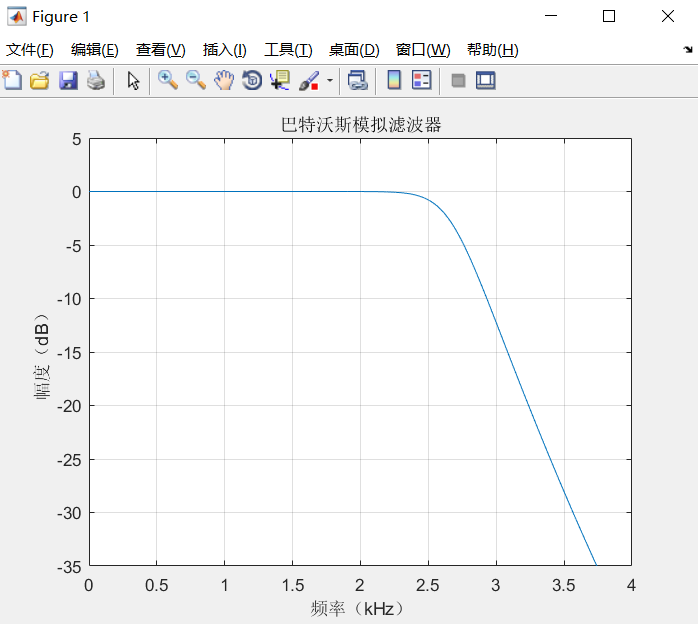图3.1.4响应特性对于巴特沃斯低通滤波器的显示
验证结果
经仿真得到结果：
巴特沃斯滤波器随着频率升高幅度单调变化，图像平顺，波纹稳定不起伏。完全符合巴特沃斯滤波器特点，实例仿真成功。
4总结
Matlab的运用大大简化了设计过程，降低了设计成本，不仅按照要求设计出了满足指标的巴特沃斯滤波器，还通过波特图显示出了其幅频曲线，严谨的事实依据为其验证提高了可靠性，体现了科学性，推动了巴特沃斯滤波器的各项研究进程，为以后深入研究打下了牢固基础。
参考文献：
吴忻生，唐萍，秦瀚.数字滤波技术在称重系统信号采集中的应用[J].传感器与微系统，2010，29(09)：131-134.
张小虹.数字信号处理[M].北京：机械工业出版社，2008.

展开全文• 用双线性变换法设计一个巴特沃斯低通IIR数字滤波器。设计指标参数为：在通带内频率低于0.2π时，最大衰减问题补充：用matlab程序写，谢谢大家●fp=100HZ,fs=300HZ，αp=3dB,αs=20dB,抽样频率为Fs=1000HZfp=100;fs=...
• 1. 设计IIR巴特沃斯低通滤波器 2. 将滤波器用于加噪声信号的处理 3. 改变参数指标比较不同 4. 实验结果与分析 （1）滤波器频率特性如图 输入信号特性如图: 经过滤波器之后信号特性如图: ...DSP
• 本次设计围绕四阶巴特沃斯低通滤波器，从电路设计，时域分析，频率分析，S域分析几个方面着手，通过理论分析、仿真实验、真实测量来研究和验证整个系统的性质。在时域上验证系统的冲激响应和阶跃响应以及零输入响应...
• %得出模拟滤波器系数 figure freqs(B,A) [Bz,Az]=bilinear(B,A,fs);%利用双线性变化法转换成数字滤波器 figure freqz(Bz,Az); [H,w]=freqz(Bz,Az);%得出函数值 subplot(211) hold on plot([0.35,0.35],[0,-300],':g...
• 基于matlab的IIR巴特沃斯滤波器设计，利用双线性变换法转换模拟到数字。
• 对传感器测量的数据加了噪声，然后对其加了巴特沃斯低通滤波，利用正则化方法进行数据的逆推，对逆推的结果又加了一次滤波，发现出现了时间位移，找了半天资料也不知道如何解决，希望有懂的人可以指导一下，具体程序...测量噪声 matlab
• 本题选择IIR巴特沃斯低通滤波器，设计一个IIR滤波器需要一下步骤： a. 根据技术指标通带截止频率fs、阻带截止频率fp，通带最大衰减ap、 阻带最小衰减as，求出滤波器的阶数N。 b.求出归一化极点pk，将pk代入，得到...数字信号处理
• 可以运行，本人的大作业，处理的彩色图片，对三通道分别处理MATLAB
• 因为教研室项目要求，近期做了关于巴特沃斯滤波器部分，采用的是C#与Matlab混合编程的方式，由于是第一次写博客，还有许多不足的地方。教研室用的VS版本为2017版，而MatlabR2012a和MatlabR2014b似乎并不支持VS2017版...
• 最近学到第六章了，利用模拟低通滤波器设计不同类型的模拟滤波器，我们重点在设计巴特沃斯滤波器。 巴特沃斯滤波器通带、阻带具有单调下降的幅频特征，过渡带在与切比雪夫、椭圆滤波器相比中最宽。 利用高通到低通、...带阻滤波器 带通滤波器 高通滤波器
• 滤波器设计、低通滤波器巴特沃斯滤波器滤波器设计、低通滤波器巴特沃斯滤波器
• 无限脉冲响应数字滤波器设计 ...低通 高通 全通 带通 带阻 二、数字滤波器的设计方法 1、按要求确定滤波器的性能指标 2、用IIR或FIR系统函数去逼近这一性能要求 3、选择适当的运算结构去实现这个系统函数 4、数字信号处理
• 院系电气信息工程学院 姓名 XXX 学号 XX 专业 XXXXXX 课程设计题目 基于MATLAB设计巴特沃斯低通滤波器 指导教师 XX 主要内容: 本文重点研究怎样通过MATLAB来模拟巴特沃斯低通滤波器讨论巴特沃斯低通滤波器在实际中...
• 通信系统仿真课程设计任务书 院系电气信息工程学院 XXX 学号 XX 专业 XXXXXX 课程设计题目 基于 MATLAB 设计巴特沃斯低通滤波器 指导教师 XX 主要容 : 本文重点研究怎样通过 MATLAB 来模拟巴特沃斯低通滤波器讨论...
• 四旋翼惯导融合之观测传感器滞后问题汇总与巴特沃斯低通滤波器设计（气压计MS5611、GPS模块M8N、超声波、PX4FLOW等）
• 1、背景对于微弱的信号的处理方式一般是：放大和滤波，这个过程中就涉及到放大电路的选取、滤波器的选择以及偏置电路的设计。本例以实例的方式讲解并附带参数计算、仿真、实物测试三个环节。假设需要处理一个20mV的...
• 我的采样周期是250hz，截止频率是20hz，用的2阶巴特沃斯滤波器，已经用matlab把相位延时的图做出来了，有大神知道怎么算出10hz时对应的延时时间是多少毫秒?信号与系统 滤波算法
• 1、背景对于微弱的信号的处理方式一般是：放大和滤波，这个过程中就涉及到放大电路的选取、滤波器的选择以及偏置电路的设计。本例以实例的方式讲解并附带参数计算、仿真、实物测试三个环节。假设需要处理一个20mV的...
• 这意味着 信号的模拟频率 fff= 50 (Hz)，注意它的单位是Hz 信号的表达式为 y=sin(2πft)=sin(2π∗50t)=sin(100πt)y = sin(2\pi ft)=sin(2\pi *50 t)=sin(100\pi t)y=sin(2πft)=sin(2π∗50t)=sin(100πt) 由于...matlab...